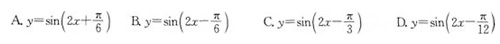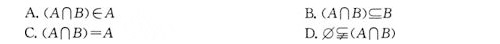﻿ 成考高起点理工类数学模拟练习题_大牛教育成考网

# 成考高起点理工类数学模拟练习题

数学是一门可以有很大跨度的学科，只要成考生能掌握好教材的知识点，加以运用，辅之于平时的练习，成绩有一个很大的提高是不难的。以下是成人高考高中起点理工类《数学》科目的模拟练习题，供考生参考。

1、函数y=log5x(x>0)的反函数为(　　)

A.y=x5(x∈R)

B.y=5x(x∈ R)

C.y=5x(x∈R)

D.y=1/5x(x∈R)

2.在△ABC中，∠C=30o，则cos Acos B-sin Asin B的值等于(　　)3、把函数y=sin2x的图象向右平移詈个单位，所得函数的解析式为(　　)4、设定义在R上的函数?(x)=x|x|，则(x)是(　　)

A.奇函数，又是增函数

B.偶函数，又是增函数

C.奇函数，又是减函数

D.偶函数，又是减函数

5、不等式ax2+bx+24<0的解集为x>2或x<-4，则(　　)

A.a=-3，b=-6

B.a=3，b=-6

C.a=-3，b=6

D.a=3，b=6

6、对任意两个集合A，B，下列命题中正确的是(　　)﻿

### 院校导航﻿